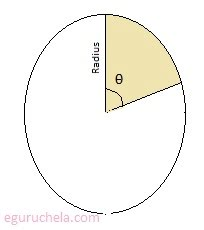### Find area of sector of circle

The area of sector can be calculated for given value which can be in radian or in degree. one degree is equal to 0.0174533 radian (approx), simlarlly one radian is equal to 57.2958 degree (approx). The fraction is determined by the ratio of the arc length to the entire circumference.
 Please enter R (Radius of Circle) = Please enter SA (Sector Angle) = in radians/degrees Area of Sector (If angle given in radians) = Area of Sector (If angle given in degrees) =$$\text {The area of the circle } = \pi \times radius^{2}$$ $$\text {and the circumference circle} = 2 \pi \times radius$$ Now we can use of the angle is clearly delineated, therefore we can replace once around (2π) with the subtended angle and we get the formula we needed for the sector as follows: $$Area = (\frac {Angle}{2}) \times radius^{2}$$ Let's take the example : radius is 7 cm and angle is 60o, now we can convert the 60o into radian value as follow:
Since 360o in radian = 2π, therefore 60o in radian = 60 * (2/360) = 1/3π = 0.3333π.
= 0.33333 X 3.141592654 = 1.047619
We can enter the value in both form as degree (60) or as radian (1.047619).
The area of sector can be calculated using following formula $$\text {Area of sector = } \pi r^{2} \cdot \frac {\theta^{o}}{360}$$ where π is 3.141592654, r is radius of thr circle and θ is angle in degree